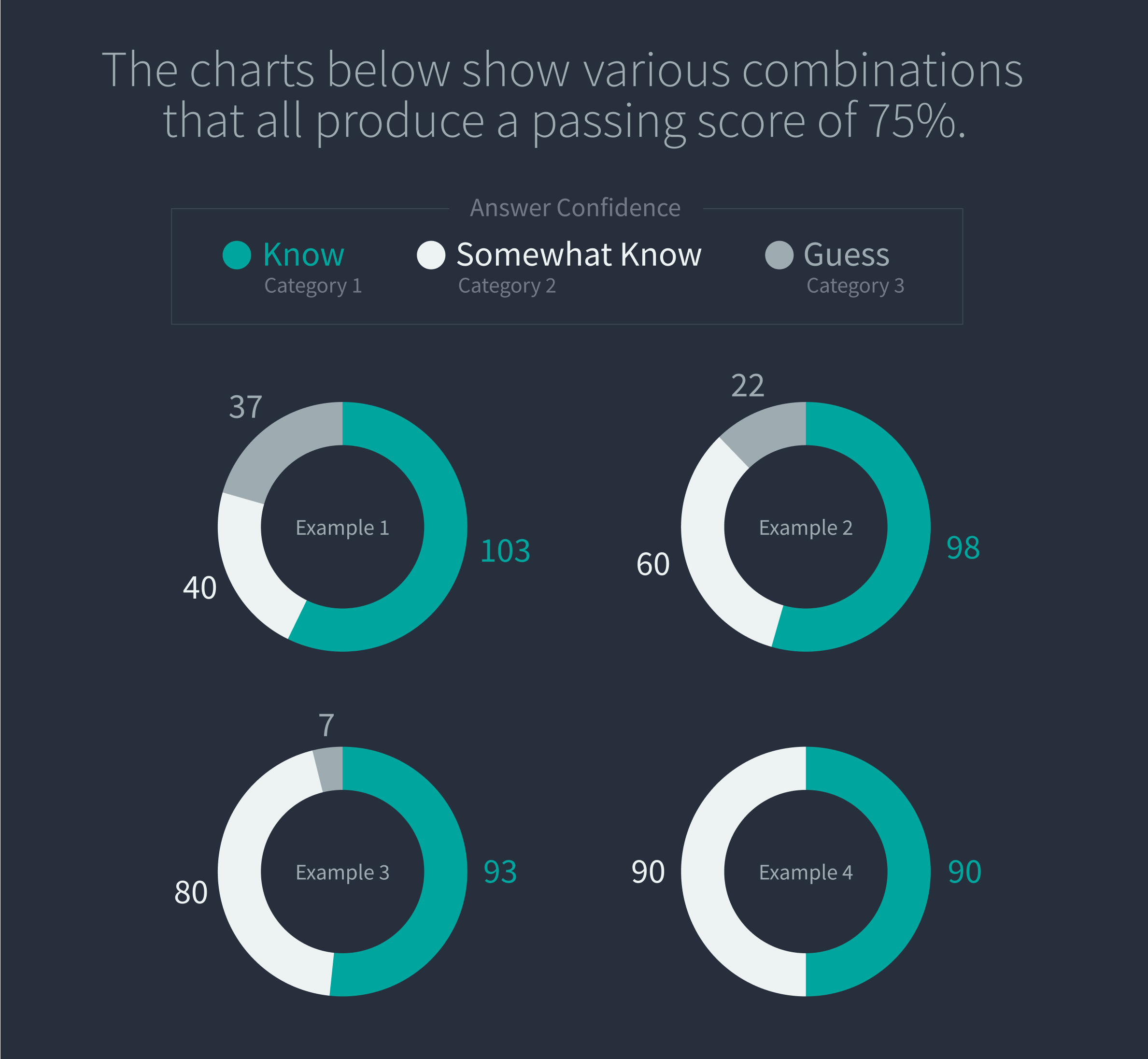# Strategies for Passing the CFA Level I Exam

by Tong Teh, CFA, FSA

You’re dedicating yourself to studying, but what is your target? The better you know your target, the more productive your studying will be and the higher your chances of success.

## Focus on What You Can Accomplish on the CFA Exam

The key to passing CFA Level I is to answer all the questions you know you can answer correctly. There are a total of 180 questions in the CFA Level I exam. The exam is composed of 90 questions in the first session and 90 questions in the second session. To pass this exam, aim for correctly answering at least 75% of the questions. That means you need to correctly answer at least 180(0.75) = 135 questions total, or about 68 questions in each session. The key is to identify these 68 questions in each session of your exam and solve them correctly.

When you are going through the exam, you must be willing to skip a problem. There is no point in spending too much time on a single problem, then ending up with insufficient time, leading you to make careless mistakes on easier problems.

Keep in mind that all questions in the multiple-choice exams count the same. Solving a very easy problem correctly and solving a very difficult problem correctly result in the same amount of points. Remember that your goal in the exam is to answer enough questions correctly to pass. Why bother spending 3 minutes solving a difficult question (which you may end up not being able to solve anyway) when you can use those 3 minutes to solve 2 or more easier questions?

So, what does this mean? You should spend the first 10 seconds of every question making an assessment:

• If you don’t understand the problem during the first read, skip it.
• If you think that you may be able to do the problem but may take some time to come up with the solution, mark it and skip it.
• If you understand the question and think you can solve it quickly, solve it.

Apply this strategy to all the questions during your first round through the exam. After that, come back to the questions you’ve marked and attempt those questions in your second round. Finally, answer the questions you have skipped by making your best guess, which could be a random guess.

If you’re comfortable with at least 68 questions out of the 90 problems in each session, you should be able to pass the exam! In reality, you will likely need to know the answer to fewer than 68 questions out of the 90 questions to pass the exam.

## Passing the CFA Exam Might Be Easier Than You Think

What score do you need to pass the CFA Level I exam? Unfortunately, the CFA Institute doesn’t give an exact number. They do give enough information to determine a reasonable estimate, but rather than debate the unknowable, we are going to assume a 75% passing score. It is on the conservative side, but not by much.

If 75% is required to pass, then you need to answer 135 questions correctly. You may think you need to know the answer to 135 of the questions, but that is not the case. You really need to know the answer to fewer than 135 questions, as we will illustrate below.

## First, let’s divide the exam questions into 3 categories:

1. For Category 1 questions, you know the answer. You love these questions and feel confident when you’re answering.
2. For Category 2 questions, you’re not quite sure of the answer, but you can eliminate one of the options. You might spend a lot of time on these questions because you are familiar with the topic but can’t quite determine the answer.
3. For Category 3 questions, you have no idea, so you must randomly guess. These are the ones you think come from some other syllabus!

## Second, consider the probability you’ll answer these questions correctly.

Now let’s think about the probability of getting the correct answer for each question category. Since you know the answer for Category 1, you have a 100% chance of getting it right. For Category 2, let’s use 50%, which is consistent with being able to eliminate one of the three choices. Finally, answers to Category 3 questions have just a 33% chance of being correct.

Remember you need 135 correct questions to pass. You can determine how many questions you must know (Category 1) if you assume how many questions fall into Category 2. For example, assume 60 out of the 180 questions fall into Category 2. That means you will be familiar with a third of the questions but not know the answer for sure.

Then you can solve for how many questions you need to know with the following formula:

• 135 = (100%)(Category 1) + (50%)(Category 2) + (33%)(Category 3)
• 135 = (100%)(Category 1) + (50%)(60) + (33%)(180 ­– 60 – Category 1)
• Category 1 = {135 – (50%)(60) – (33%)(180 – 60)} / 0.67 = 98

If you somewhat know 60 of the 180 questions, you only need to know 98 of the 180 questions for sure. That means you can randomly guess on 22 questions.## What does this mean for you?

If you need to correctly answer 135 questions to pass the exam, you don’t have to know the answer to 135 questions. That should give you confidence. It’s OK to guess on some questions. A passing score is within your grasp!